# SPC model of water

(diff) ← Older revision | Latest revision (diff) | Newer revision → (diff)

The simple point charge (SPC) model  is an empirical model of water. The molecule is modelled as a rigid isosceles triangle, having charges situated on each of the three atoms. As well as Coulombic interactions, the molecules interact via long-range Lennard-Jones sites, situated on the oxygen atoms. The parameters are as follows:

 parameter value$\sigma$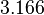$3.166$Å$\epsilon$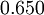$0.650$ kJ mol-1$r_\mathrm{OH}$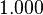$1.000$Å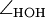$\angle_\mathrm{HOH}$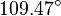$109.47^{\circ}$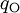$q_{\mathrm{O}}$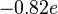$-0.82 e$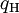$q_{\mathrm{H}}$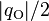$|q_{\mathrm{O}}|/2$ (charge neutrality)

The SPC model has a dipole moment of 2.27 D.

## Surface tension

The surface tension has been studied for the SPC model by Vega and Miguel. 

## Related models

Over the years a number of variants of the SPC model have been published: